# Mathematics 1010 online

## Translating and Scaling Graphs

If you see this red text then unfortunately your browser does not support applets. You may have to enable Java, or use a different system.Click here to see a gif image of the applet that you should be seeing.

The applet just above this paragraph on this page can help you understand the effects of simple shifts (or translations) and rescalings of a graph. You can either look at the graph and deduce the equation, or start with the equation and figure out the graph. There is a detailed explanation in terms of parabolas. Here we give a summary in terms of a general function, and then explain the operation of the applet.

Suppose we start with a basic functionand consider its graph, i.e., the graph of the equation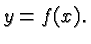This is the basic graph and you can have the applet display it for the following special choices of. Click on the description of the function to see its graph. (Use the Back button of your browser to return here.)

Consider now the functionfor some constants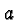,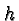, and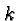. Naturally, the graph of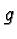will be similar to that of. Specifically, the graph ofis obtained from that ofby
1. Shifting the graph ofunits to the right. This is a horizontal translation of the graph.
2. Multiplying the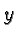value of the shifted graph by. This is a scaling (or rescaling), of the graph.
3. Translating the shifted and scaled graph up byunits. This is a vertical translation of the graph.

These ideas are illustrated for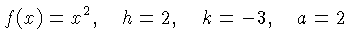in the nearby Figure.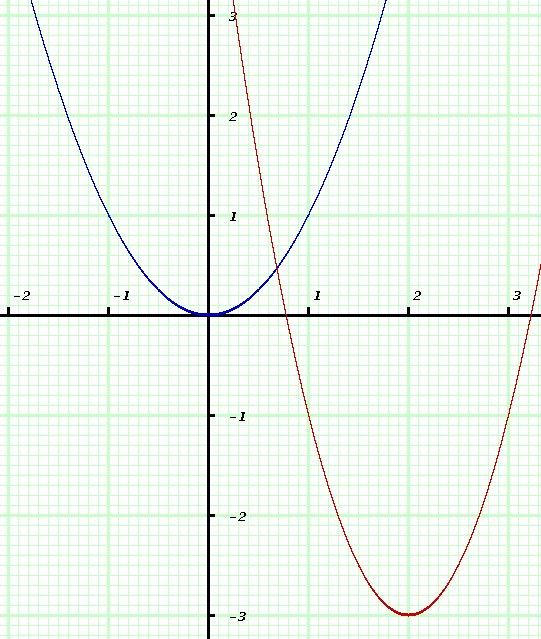Figure 1: An Example.

The blue graph is the graph of. The red graph is the graph of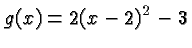corresponding to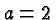,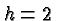, and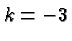.

This Figure was created with the applet on this page. The remainder of the page describes how to operate the applet.

### Operating the applet.

In what follows we'll refer to the values of,andas the data and to the graph of the corresponding function simply as the graph or the modified graph.

To use the software, follow these steps:

• Click on the applet on this page, where it says to click. If your browser does not support java code then a message will appear at the top of this page telling you so, and inviting you to view a picture of what you want to be seeing instead of that message.

• Two windows will appear on your screen. One is a control window with buttons to click, and places to enter data. The other shows a parabola drawn in a coordinate system that is imposed on a fine grid resembling Engineering graph paper. The parabola is the graph of the default basic function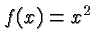. The basic function is always drawn in blue. The graph corresponding to the data is drawn in red. A graph corresponding to data specified by you is drawn in magenta (purple).

• You can operate the software in various modes:
1. Given the data draw the graph.
2. Given the graph, figure out the data.
3. Specify the data and see the corresponding graph.

• The buttons on the Control Panel have the following effects:
• Quit finishes your session and causes the windows to disappear.
• New creates a new data set and corresponding graph.
• Show shows the current data and the graph.
• Erase erases the graph (in mode 1) or the data you entered (in mode 2).
• Graph or Algebra toggles between modes 1 and 2.
• Basic toggles on and off the drawing of the basic function. You usually want to see the basic function so you know what you are working with, but if you wish to spice up things you can turn the basic function off.
• Guess causes the graph of the function to be drawn that corresponds to the current data in the text fields of the control panel. If no data exist, the defaults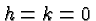and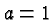(corresponding to the basic function) are used.
• Scale Toggles between 50 and 100 pixels per unit length. 100 pixels look nicer, but you may have to use 50 (the default) to show all the relevant parts of all graphs. You may be able to resize your drawing window and use the larger scale.

• The drop down menu in the control panel lets you select a new basic function from those described above.

• The three blue text fields show the current data, or let you enter them. To enter a number, click on the text field, type the number, and hit enter. If the code cannot understand what your are typing (for example if you type the phrase "the secret value") it will use a default value instead.

• To determine the graph do as follows:
1. Select mode 1 by clicking on the button labeled Graph if that label is present.

2. Focus on the point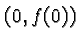on the graph of the basic function. For all but the last function this is the origin. For the bell shaped function this is the point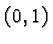.

3. Figure out the corresponding point on the modified graph. Locate that point in the drawing window and click on that point. You need to be within 12 pixels of that point.

4. Find any other point on the graph more than one unit away on the first point and click on it.

5. If all went well the modified graph will appear and you can be proud of yourself. If something went wrong the two points you clicked on will disappear and you can try again. If you make a mistake and click on the wrong point you can use the Erase button to undo your action.

• To determine the data proceed as follows:
1. Select mode 2 by clicking on the button labeled Algebra if that label is present.

2. Look at the graph in the drawing window and figure out the data.

3. Enter them in the text fields. If you enter the correct values the corresponding text fields will change color.

4. To see the graph corresponding to the data you have so far entered click on the button labeled Guess.

5. Start with a simple function, i.e.,or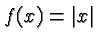and go on to the other functions only after you gain experience. The last, bell shaped, function is much more challenging than the other functions.

6. When all three text windows have changed color you have succeeded and you can again feel proud.

• To see various graphs select mode 1 and click repeatedly on New. To see the data corresponding to a particular function click on Show.